# Catch up on the latest AI articles# Synthetic Image Generation By Combining Diffusion Models

3 main points
✔️ We proposed a method to generate synthetic images using a diffusion model.
✔️ The diffusion model was combined by viewing it as an energy-based model and allowing it to be combined across different domains using logical products and negations.
✔️ Experimented on multiple data sets and outperformed the baseline model quantitatively and qualitatively.

Compositional Visual Generation with Composable Diffusion Models
written by Nan LiuShuang LiYilun DuAntonio TorralbaJoshua B. Tenenbaum
(Submitted on 3 Jun 2022 (v1), last revised 17 Jan 2023 (this version, v6))
Comments: ECCV 2022. First three authors contributed equally.

Subjects:
Computer Vision and Pattern Recognition (cs.CV)

The images used in this article are from the paper, the introductory slides, or were created based on them.

## Introduction

Language input-type diffusion models such as DALLE-2 can generate very elaborate images from natural language sentences. Still, they are not sufficient to understand combinations of concepts such as relations between objects. Therefore, this paper proposes a method to combine diffusion models to generate synthetic images.

## technique

### Noise reduction by the diffusion model

Denoising Diffusion Probabilistic Models (DDPMs) are a type of generative model that removes noise. Assuming that the output image ${\bf x}_0$ is generated in $T$ steps from the noise ${\bf x}_T$ created from a Gaussian distribution, the forward process of noise multiplication $q({\bf x}_t|{\bf x}_{t-1})$ and reverse process of noise removal $p({\bf x}_{t -1}|{\bf x}_{t})$ to remove noise is as follows.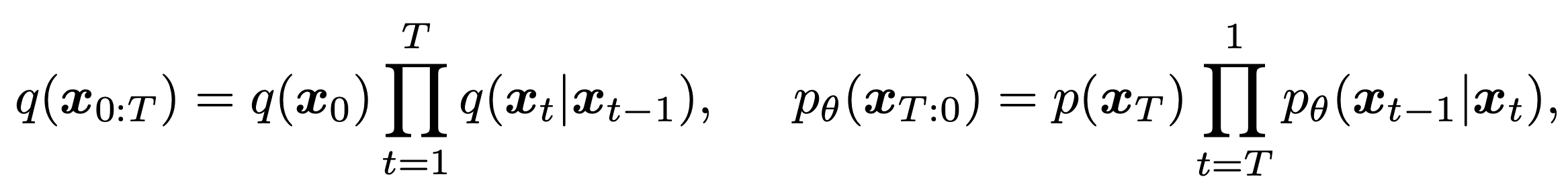where $q({\bf x}_0)$ is the true data distribution and $p({\bf x}_T)$ is the normal Gaussian distribution. The generative process $p_{\theta}({\bf x}_{t-1}|{\bf x}_t)$ learns to approximate the reverse process to produce the true image. Each generating process learns the mean and variance parameters and is represented as follows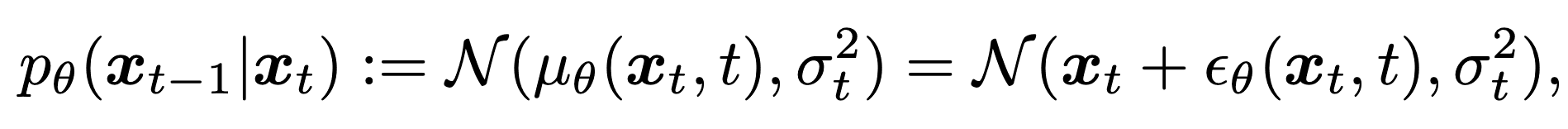That is, ${\bf x}_{t-1}$ is the noisier ${\bf x}_t$ plus the perturbation term ${\epsilon}_{\theta}({\bf x}_t,t)$ by the mean $\mu_\theta({\bf x}_t,t)$ and variance ${\sigma}_t$. It is parametrized and expressed as

### an energy-based model

Energy-based models (EBMs) are a type of generative model in which the image ${\bf x}\in {\mathbb R}^D$ is represented by a denormalized probability density such that

$$p_\theta({\bf x})\propto e^{-E_\theta({\bf x})}$$

where $E_\theta({\bf x})$ is a neural network. In the gradient-based MCMC process, the generated image is refined using Langevin dynamics as follows

$${\bf x}_t={\bf x}_{t-1}-\frac{\lambda}{2}\nabla_{\bf x}E_\theta({\bf x}_{t-1})+{\mathcal N}(0,\sigma^2)$$

Comparing the above equation with the equation for the generation process in the diffusion model, we can see that they are equatorially similar.

### Diffusion model as an energy-based model

As mentioned earlier, the diffusion model learns a noise reduction network $\epsilon_\theta({\bf x}_t,t)$ to generate the images, and the EBMs are generated through $\nabla_{\bf x}E_\theta({\bf x}_t)\propto \nabla_{\bf x}\log p_\theta(\bf x)$. Since both terms are due to the scores of the data distribution, they can be seen as mathematically equivalent. Thus, the EBMs can be combined and applied to the diffusion model.

Now, given $n$ independent EBMs $E^1_\theta({\bf x}),\cdots ,E^n_\theta({\bf x})$, we can combine them to obtain a new EBM as follows

$$p_{compose}({\bf x})\propto p_{\theta}^1({\bf x})\cdots p_\theta^n({\bf x})\propto e^{-\Sigma_iE_\theta^i({\bf x})}=e^{-E_\theta({\bf x })}$$

where $p_\theta^i$ is the probability density of ${\bf x}$. The generated image will look like this

$${\bf x}_t={\bf x}_{t-1}-\frac{\lambda}{2}\nabla_{\bf x}Sigma_iE_\theta^i({\bf x}_{t-1})+{\mathcal N}(0,\sigma^2)$$

Similarly, the generation process in the diffusion model can be expressed as follows

$$p_{compose}({\bf x}_{t-1}|{\bf x}_t)=\mathcal N({\bf x}_t+\Sigma_i \epsilon^i_\theta({\bf x}_t,t),\sigma_t^2)$$

The diffusion model can be combined by parametrizing $\epsilon_\theta({\bf x},t)$ as the gradient of the EBM.

### composite image generation

To generate a composite image, we combine diffusion models, each with an independent concept ${\bf c}_i$. The operators used are logical conjunction ( AND) and negation ( NOT).

AND

$$p({\bf x}|{\bf c}_1,\cdots ,{\bf c}_n)\propto p({\bf x},{\bf c}_1,\cdots ,{\bf c}_n)=p({\bf x})\Pi_i p({\bf c}_i|{\bf x})=p({\bf x})\Pi_i\frac {p({\bf x}|{\bf c}_i)}{p({\bf x})}$$

Substituting the expression for the generation process obtained above, we obtain the following equation

$$p^*({\bf x}_{t-1}|{\bf x}_t):=\mathcal N({\bf x}_t+\epsilon^*({\bf x}_t,t),\sigma_t^2),$$

$$\epsilon^*({\bf x}_t,t)=\epsilon_\theta({\bf x}_t,t)+\alpha \Sigma_i(\epsilon_\theta({\bf x}_t,t|{\bf c}_i)-\epsilon_\theta({\bf x}_t, t))$$

where $\alpha$ is the temperature parameter.

NOT

The concept we want to negate as $\tilde{{\bf c}}_j$.

$$p({\bf x}|not\ \tilde{{\bf c}_j},{\bf c_1},\cdots ,{\bf c}_n)\propto p({\bf x}, not\ \tilde{{\bf c}_j},{\bf c}_1,\cdots, {\bf c}_n)=p({\bf x})\frac{\Pi_i p({\bf c}_i|{\bf x})}{p(\tilde{{\bf c}_j}|{\bf x})^\beta}=p({\bf x})\frac{p({\bf x})^\beta}{p({\bf x}|\tilde{{\bf c}_j})^\beta}\Pi_i\frac{p({\bf x}|{\bf c}_i)}{p({\bf x})}$$

$$\epsilon^*({\bf x}_t,t)=\epsilon_\theta({\bf x}_t,t)+\alpha\{-\beta(\epsilon_\theta({\bf x}_t,t|\tilde{\bf c}_j)-\epsilon_\theta({\ bf x}_t,t))+\Sigma_i(\epsilon_\theta({\bf x}_t,t|{\bf c}_i)-\epsilon_\theta({\bf x}_t,t))\}$$

where $\alpha,\beta$ are temperature parameters.

## result

Images were generated and evaluated for different domain datasets with this method and the baseline model.

### natural language binding

We compared the results of generating a combination of natural language sentences using the trained GLIDE model. It can be seen that our method generates more accurate images, such as the first one "polar bear" and the third one "bangalows".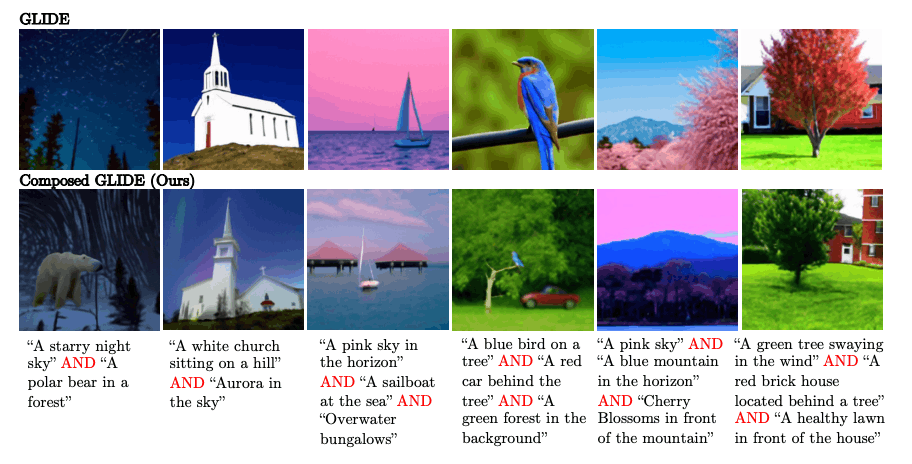### object association

The image is generated from the two-dimensional location of the object. From the figure below, it can be seen that this method correctly generates even those that made mistakes in the baseline model. Also, from the table below, both Acc and FID have been significantly updated.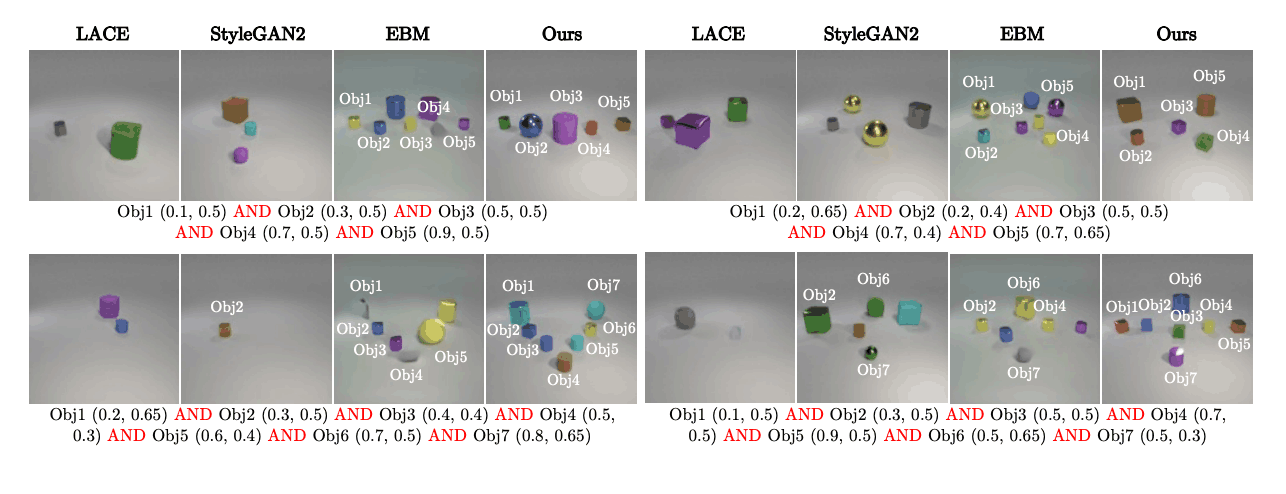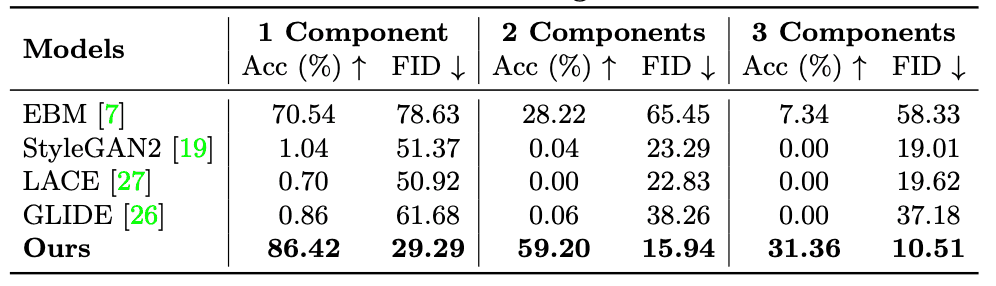### object relations

The image is generated from a sentence describing the positional relationship between objects. The figure below shows that the EBM and this method reproduce well. In addition, the table below shows that this method has a much higher Acc than the models excluding EBM while maintaining a low FID, which would be higher with EBM.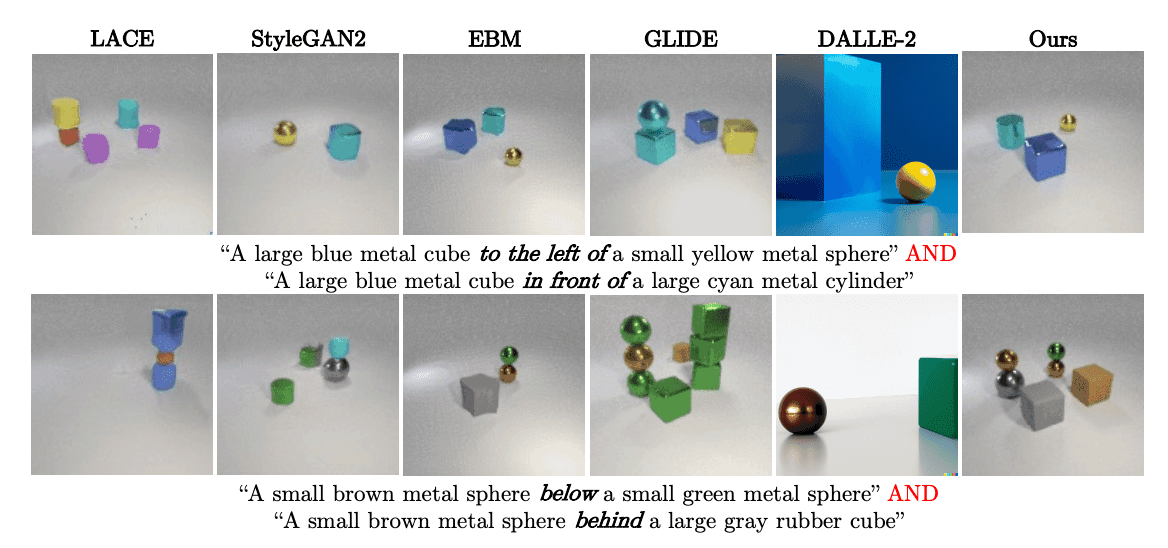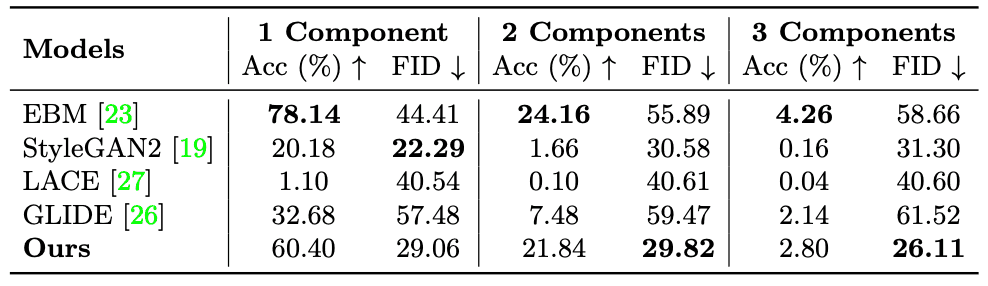## summary

In this paper, we generated images by combining diffusion models. The results show that by viewing the diffusion model as an energy-based model, it successfully generates images combining complex concepts with high accuracy. One limitation is that combining diffusion models trained on different datasets may fail, which could be improved by better implementation of the EBM structure.

If you have any suggestions for improvement of the content of the article,